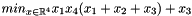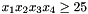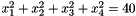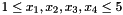Quick start

Solving a problem is done through several steps:

• Define your cost function by deriving one kind of function, depending on whether or not you want to provide a jacobian and/or a hessian.
• Define your constraints functions in the same manner.
• Build an instance of problem matching your requirements.
• Use one of the solvers to solve your problem.

The following example defines a cost function F and two constraints G0 and G1.

## Problem definition

The problem that will be solved in this tutorial is the 71th problem of Hock-Schittkowski:with the following constraints:

•••## Defining the cost function.

The library contains the following hierarchy of functions:

These types correspond to dense vectors and matrices relying on Eigen. RobOptim also support dense matrices and you can even extend the framework to support your own types.

When defining a new function, you have to derive your new function from one of those classes. Depending on the class you derive from, you will be have to implement one or several methods:

• impl_compute that returns the function's result has to be defined for all functions.
• impl_gradient which returns the function's gradient is to be defined for DerivableFunction and its subclasses.
• impl_hessian for TwiceDerivableFunction functions and its subclasses.

It is usually recommended to derive from the deepest possible class of the hierarchy (deriving from TwiceDerivableFunction is better than DerivableFunction).

Keep in mind that the type of the function represents the amount of information the solver will get, not the real nature of a function (it is possible to avoid defining a hessian by deriving from DerivableFunction, even if you function can be derived twice).

In the following sample, a TwiceDerivableFunction will be defined.

struct F : public TwiceDerivableFunction
{
F () : TwiceDerivableFunction (4, 1)
{
}

void
impl_compute (result_t& result, const argument_t& x) const throw ()
{
result (0) = x * x * (x + x + x) + x;
}

void
{
grad = x * x + x * (x + x + x);
grad = x * x + 1;
grad = x * (x + x + x);
}

void
impl_hessian (hessian_t& h, const argument_t& x, int) const throw ()

{
h (0, 0) = 2 * x;
h (0, 1) = x;
h (0, 2) = x;
h (0, 3) = 2 * x + x + x;

h (1, 0) = x;
h (1, 1) = 0.;
h (1, 2) = 0.;
h (1, 3) = x;

h (2, 0) = x;
h (2, 1) = 0.;
h (2, 2) = 0.;
h (2, 3) = x;

h (3, 0) = 2 * x + x + x;
h (3, 1) = x;
h (3, 2) = x;
h (3, 3) = 0.;
return h;
}
};


## Defining the constraints.

A constraint is no different from a cost function and can be defined in the same way than a cost function.

The following sample defines two constraints which are twice derivable functions.

struct G0 : public TwiceDerivableFunction
{
G0 ()
: TwiceDerivableFunction (4, 1)
{
}

void
impl_compute (result_t& result, const argument_t& x) const throw ()
{
result (0) = x * x * x * x;
}

void
{
grad = x * x * x;
grad = x * x * x;
grad = x * x * x;
grad = x * x * x;
}

void
impl_hessian (hessian_t& h, const argument_t& x, int) const throw ()
{
h (0, 0) = 0.;
h (0, 1) = x * x;
h (0, 2) = x * x;
h (0, 3) = x * x;

h (1, 0) = x * x;
h (1, 1) = 0.;
h (1, 2) = x * x;
h (1, 3) = x * x;

h (2, 0) = x * x;
h (2, 1) = x * x;
h (2, 2) = 0.;
h (2, 3) = x * x;

h (3, 0) = x * x;
h (3, 1) = x * x;
h (3, 2) = x * x;
h (3, 3) = 0.;
}
};

struct G1 : public TwiceDerivableFunction
{
G1 ()
: TwiceDerivableFunction (4, 1)
{
}

void
impl_compute (result_t& result, const argument_t& x) const throw ()
{
result (0) = x*x + x*x + x*x + x*x;
}

void
{
}

void
impl_hessian (hessian_t& h, const argument_t& x, int) const throw ()
{
h (0, 0) = 2.;
h (0, 1) = 0.;
h (0, 2) = 0.;
h (0, 3) = 0.;

h (1, 0) = 0.;
h (1, 1) = 2.;
h (1, 2) = 0.;
h (1, 3) = 0.;

h (2, 0) = 0.;
h (2, 1) = 0.;
h (2, 2) = 2.;
h (2, 3) = 0.;

h (3, 0) = 0.;
h (3, 1) = 0.;
h (3, 2) = 0.;
h (3, 3) = 2.;
}
};


## Problem definition

The last part of this tutorial covers how to build a problem and solve it. The steps are:

• Instanciate your functions (cost functions and constraints).
• Pass them to the problem.
• Optional: set a starting point.
• Instanciate a solver which solves your class of problem.
• Solve the problem by calling minimum.
int run_test ()
{
F f;
G0 g0;
G1 g1;

solver_t::problem_t pb (f);

// Set bound for all variables.
// 1. < x_i < 5. (x_i in [1.;5.])
for (Function::size_type i = 0; i < pb.function ().n; ++i)
pb.argBounds ()[i] = T::makeBound (1., 5.);

// Set the starting point.
Function::vector_t start (pb.function ().n);
start = 1., start = 5., start = 5., start = 1.;

initialize_problem (pb, g0, g1);

// Initialize solver.

// Here we are relying on the Ipopt solver (available separately).
// You may change this string to load the solver you wish to use.
SolverFactory<solver_t> factory ("ipopt-td", problem);
solver_t& solver = factory ();

// Compute the minimum and retrieve the result.
solver_t::result_t res = solver.minimum ();

// Display solver information.
std::cout << solver << std::endl;

// Check if the minimization has succeed.
switch (solver.minimumType ())
{
case SOLVER_NO_SOLUTION:
std::cerr << "No solution." << std::endl;
return 1;
case SOLVER_ERROR:
std::cerr << "An error happened: "
<< solver.getMinimum<SolverError> ().what () << std::endl;
return 2;

case SOLVER_VALUE_WARNINGS:
{
// Get the real'' result.
Result& result = solver.getMinimum<ResultWithWarnings> ();
// Display the result.
std::cout << "A solution has been found (minor problems occurred): "
<< std::endl
<< result << std::endl;
return 0;
}
case SOLVER_VALUE:
{
// Get the real'' result.
Result& result = solver.getMinimum<Result> ();
// Display the result.
std::cout << "A solution has been found: " << std::endl;
std::cout << result << std::endl;
return 0;
}
}

// Should never happen.
assert (0);
return 42;
}


This is the last piece of code needed to instantiate and resolve an optimization problem with this package.

To see more usage examples, consider looking at the test directory of the project which contains the project's test suite.